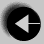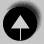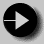## Weight Conversion with a Prompt

To illustrate this, our next program will re-use the function from 2.3. The program is similar to the last one, but instead of printing a table of weights, the user enters a weight, and this value is converted. Such a program requires user input. This can be done in two ways, both of which will be shown.

The simpler implementation is to prompt for a weight, and then read the user's keyboard input. This would be done as follows

```
#include <stdio.h>

void print_converted(int pounds)
/* Convert U.S. Weight to Imperial and International
Units. Print the results */
{       int stones = pounds / 14;
int uklbs = pounds % 14;
float kilos_per_pound = 0.45359;
float kilos = pounds * kilos_per_pound;

printf("   %3d          %2d  %2d        %6.2f\n",
pounds, stones, uklbs, kilos);
}

main()
{       int us_pounds;

printf("Give an integer weight in Pounds : ");
scanf("%d", &us_pounds);

printf(" US lbs      UK st. lbs       INT Kg\n");
print_converted(us_pounds);
}```

A printf statement is used to prompt for input. scanf is an equivalent input statement, note that the variable to be read us_pounds is written as &us_pounds here. This is very important and it will be explained later.Go to Weight conversion tableGo to IndexGo to Weight conversion with